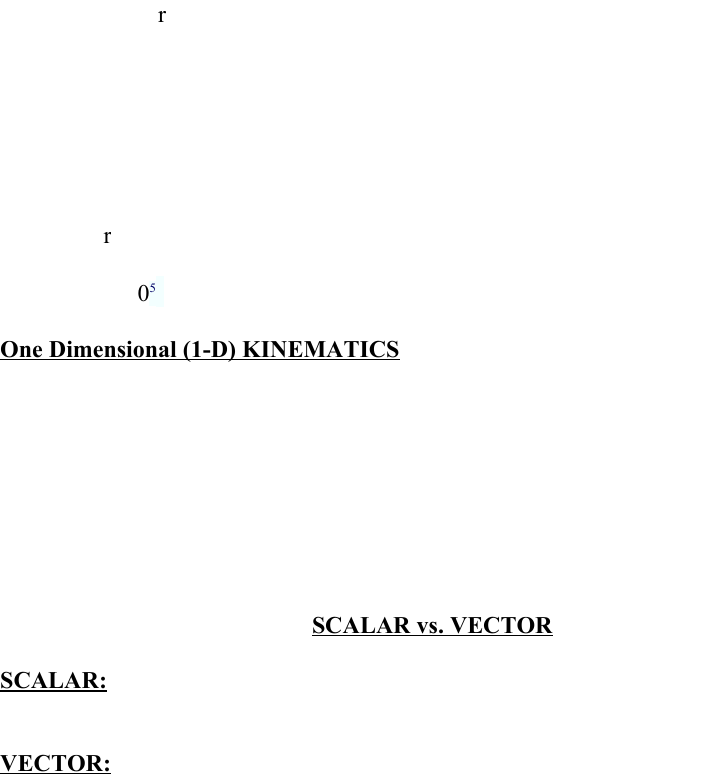Class Notes (1,100,000)
CA (620,000)
U of G (30,000)
PHYS (300)
Lecture

# PHYS 1020 Lecture Notes - Displacement (Vector), Speedometer

Department
Physics
Course Code
PHYS 1020
Professor
Melissa Williams

Page:
of 3Recap:
- Uncertainty is possible error in measurement.
- Sig digs –reliably known digits of a measurement
- Manipulation of sig digs – RULES
- Unit conversion
Reference textbook for today 1-19 to 1-20 & 2-1 to2-6
Note read text sections 1.1-1.4 not all covered In class!
Make 17.5 km/hr³ to cm/min³
1km= 1000m
100cm= 1m
60 min= 1hr
17.5 km/ hr³ X 1000m/ 1km X 100cm/ 1m X (1h/60 min) ³ = hr³/ 60³ min³
ANS 17.5 X 105 / 60³ = 8.10
One Dimensional (1-D) KINEMATICS
Kinematics- Description of Motion by use of :
- WORDS (terminology)
- DIAGRAMS (graphs)
- EQUATIONS
- Terminology (language) of motion
SCALAR vs. VECTOR
SCALAR: measured quantity that has only a size/magnitude (NO DIRECTION)
Ex. Speed, mass, distance, time, energy, temperature
VECTOR: has both magnitude and direction
Ex. How to find the athletic centre? 150m (EAST)
In Physics: the arithmetic is different when dealing with vectors compared with scalars
Ex. Distance (Scalar) vs. displacement (vector)
In 1-D no arrow needed, us + & -! To show direction.
LECTURE 3 – SEPTEMBER 16th, 2011!
Text ref: 1-19 to 1-20
Distance- how far an object moves during motion (S.I. unit metre) (SCALAR QUANT.)
Displacement- straight line from initial to final points in specified direction (m)
(VECTOR)
Displacement is a vector quantity- arithmetic (rules for addition, subtraction and
multiplication) not the sane as for scalars
- Indicates the change in position from the starting point to the final point (ie.
Straight line distance between start and finish)
Q1! : Car goes from point a –b. after it arrives at its destination, the magnitude of its
displacement is:
D) Either smaller than or equal to!
The distance the object travelled
SPEED: time rate of change of distance (m/s) (SCALAR)
VELOCITY: time rate of change of displacement (m/s) (VECTOR)
Average Speed: time rate of change of total distance, defined over a particular period of
elapsed time (SCALAR)
Instantaneous speed: speed at any instant -> Speedometer reading (SCALAR)
Average velocity- time rate of change of total distance, defined over a particular period of
elapsed time (Scalar) (total displ. Over total time)
Instantaneous Velocity: velocity at any instant -> speedometer reading but also must
include direction of motion at that moment (VECTOR)
EX. Max is driving to charlie’s house, which is 4.5km away. The speed limit is 50km/h.
if it takes max 6.0 mins to get to charlies house, does max exceed the speed limit at any
point? ANS: c) not enough info provided d/t = 4.5/ 6/60 = 45km/h = AVERAGE
SPEED) at any instant he could have been going past 50 km/h!!
Wednesday, SEPTEMBER 28 TH
slides & notes posted on courselink for weds. & Friday!! – only copied important things he said.
EX. of constant acceleration:
objects in “free fall” (ignore air resistance)
acceleration due to gravity : g= 9.8m/s2 (DOWN)
ie. all objects fall towards earth with a constant acceleration of g.
use constant acc. equations to describe motion – Replace x With Y